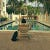# 2 Ways to Calculate Fibonacci Numbers

If you are a programming beginner or know a little bit about mathematics, you have probably heard of the Fibonacci sequence at some point. According to Wikipedia, the Fibonacci sequence is a sequence in which each number is equal to the sum of the previous two numbers.

In this blog, I will go through 2 ways you can generate this sequence using Python 3.

# Method 1: Recursion

In programming languages, recursion occurs whenever a function calls itself once or several times to compute an algorithm. You can read more about recursion here.

These are the steps to create this recursive function:

# Python Program to Validate Sudoku Solutions